RS Aggarwal Class 8 Solutions Chapter 4 - Cubes And Cube Roots

RS Aggarwal Class 8 Chapter 4 - Cubes And Cube Roots Solutions Free PDF

In this chapter, the students of class 8 will learn the basic concepts of cubes and cube roots. After solving the questions of this chapter from RS Aggarwal for class 8 the students will learn how to calculate the cube roots and cube of a given number. To help students we have also provided the RS Aggarwal class 8 solutions chapter 4 cubes and cube roots.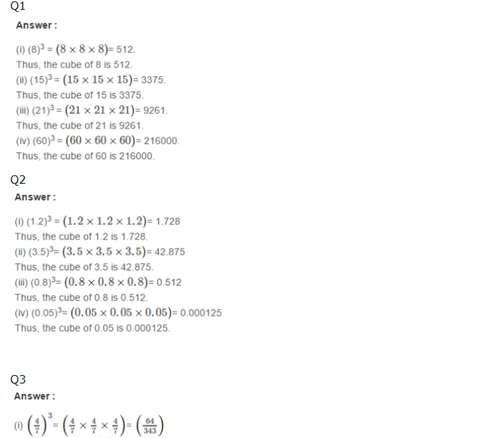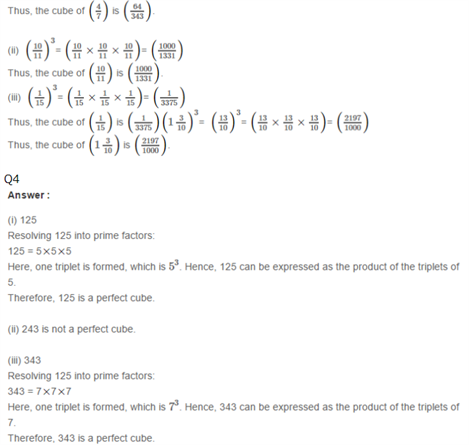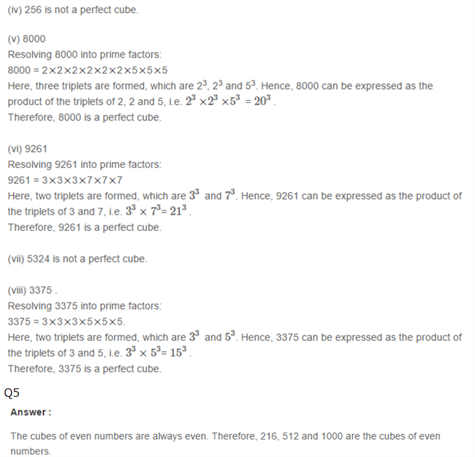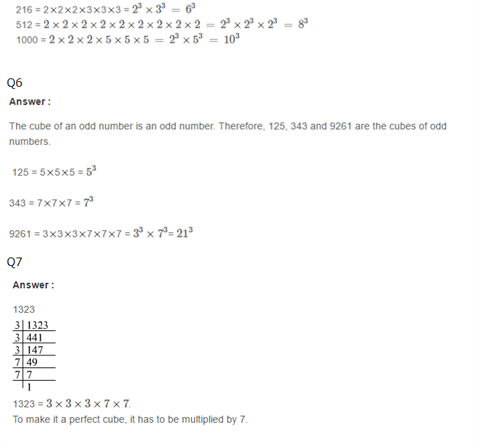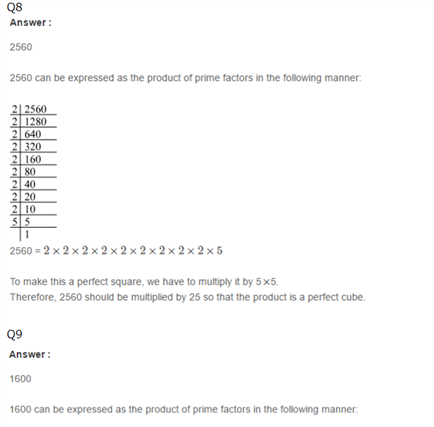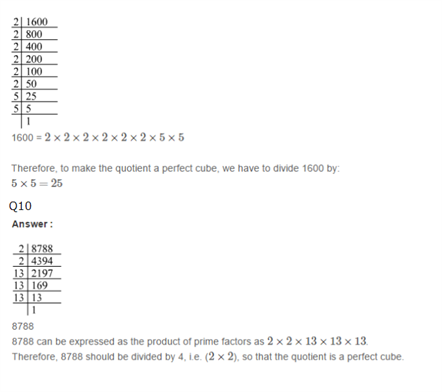Practise This Question

What are the sources of irrigation?